Скачать презентацию Advanced Graphics Geometric Methods for Ray Tracing Alex

469281197f65464ded2e312b681f5952.ppt

• Количество слайдов: 26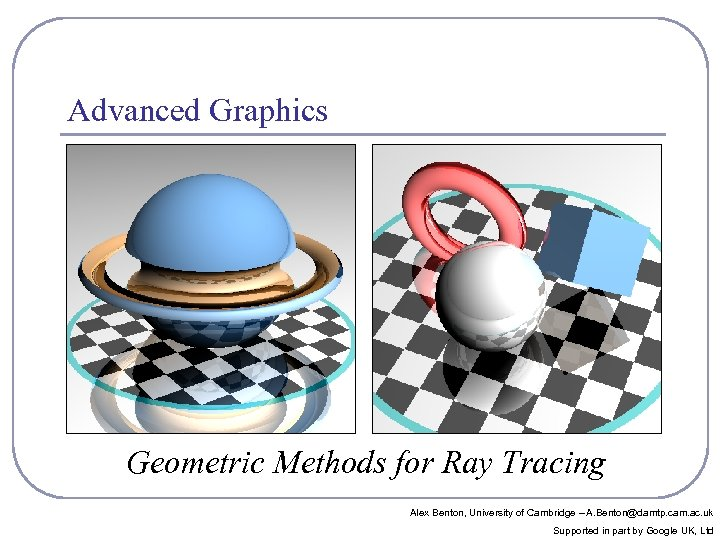Advanced Graphics Geometric Methods for Ray Tracing Alex Benton, University of Cambridge – A. [email protected] cam. ac. uk Supported in part by Google UK, Ltd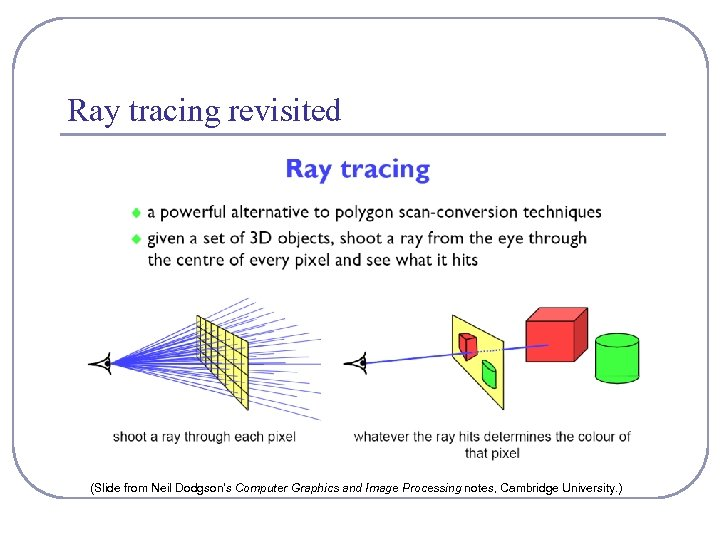Ray tracing revisited (Slide from Neil Dodgson’s Computer Graphics and Image Processing notes, Cambridge University. )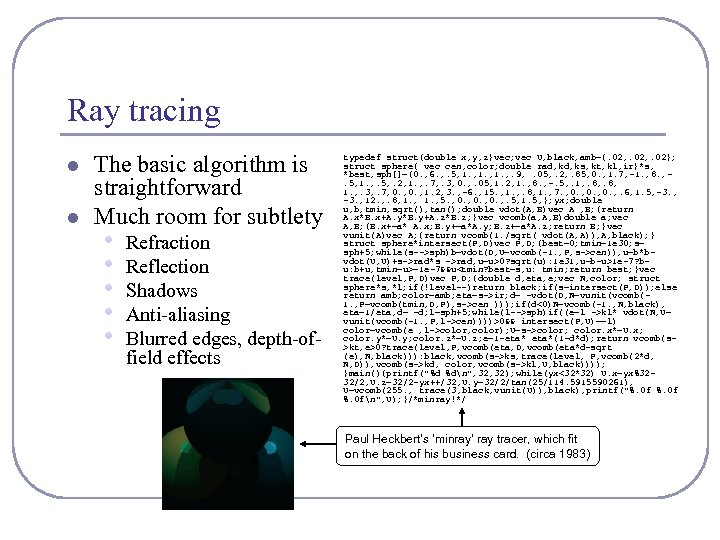Ray tracing l l The basic algorithm is straightforward Much room for subtlety • • • Refraction Reflection Shadows Anti-aliasing Blurred edges, depth-offield effects typedef struct{double x, y, z}vec; vec U, black, amb={. 02, . 02}; struct sphere{ vec cen, color; double rad, ks, kt, kl, ir}*s, *best, sph[]={0. , 6. , . 5, 1. , . 9, . 05, . 2, . 85, 0. , 1. 7, -1. , 8. , . 5, 1. , . 5, . 2, 1. , . 7, . 3, 0. , . 05, 1. 2, 1. , 8. , -. 5, . 1, . 8, 1. , . 3, . 7, 0. , 1. 2, 3. , -6. , 15. , 1. , . 8, 1. , 7. , 0. , . 6, 1. 5, -3. , 12. , . 8, 1. , 5. , 0. , . 5, 1. 5, }; yx; double u, b, tmin, sqrt(), tan(); double vdot(A, B)vec A , B; {return A. x*B. x+A. y*B. y+A. z*B. z; }vec vcomb(a, A, B)double a; vec A, B; {B. x+=a* A. x; B. y+=a*A. y; B. z+=a*A. z; return B; }vec vunit(A)vec A; {return vcomb(1. /sqrt( vdot(A, A)), A, black); } struct sphere*intersect(P, D)vec P, D; {best=0; tmin=1 e 30; s= sph+5; while(s-->sph)b=vdot(D, U=vcomb(-1. , P, s->cen)), u=b*bvdot(U, U)+s->rad*s ->rad, u=u>0? sqrt(u): 1 e 31, u=b-u>1 e-7? bu: b+u, tmin=u>=1 e-7&&uir; d= -vdot(D, N=vunit(vcomb(1. , P=vcomb(tmin, D, P), s->cen ))); if(d<0)N=vcomb(-1. , N, black), eta=1/eta, d= -d; l=sph+5; while(l-->sph)if((e=l ->kl* vdot(N, U= vunit(vcomb(-1. , P, l->cen))))>0&& intersect(P, U)==l) color=vcomb(e , l->color, color); U=s->color; color. x*=U. x; color. y*=U. y; color. z*=U. z; e=1 -eta*(1 -d*d); return vcomb(s>kt, e>0? trace(level, P, vcomb(eta, D, vcomb(eta*d-sqrt (e), N, black))): black, vcomb(s->ks, trace(level, P, vcomb(2*d, N, D)), vcomb(s->kd, color, vcomb(s->kl, U, black)))); }main(){printf("%d %dn", 32); while(yx<32*32) U. x=yx%3232/2, U. z=32/2 -yx++/32, U. y=32/2/tan(25/114. 5915590261), U=vcomb(255. , trace(3, black, vunit(U)), black), printf("%. 0 fn", U); }/*minray!*/ Paul Heckbert’s ‘minray’ ray tracer, which fit on the back of his business card. (circa 1983)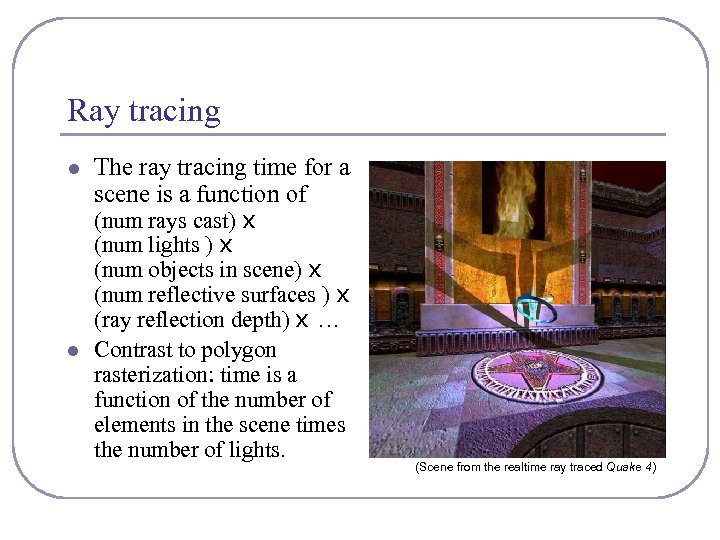Ray tracing l l The ray tracing time for a scene is a function of (num rays cast) x (num lights ) x (num objects in scene) x (num reflective surfaces ) x (ray reflection depth) x … Contrast to polygon rasterization: time is a function of the number of elements in the scene times the number of lights. (Scene from the realtime ray traced Quake 4)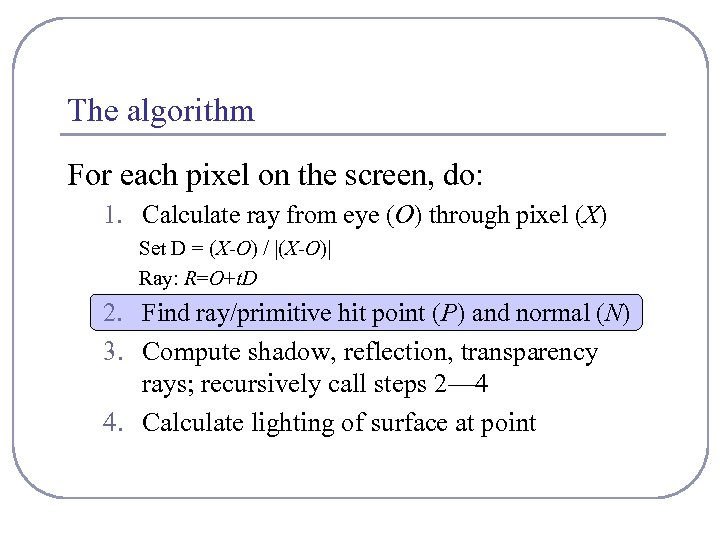The algorithm For each pixel on the screen, do: 1. Calculate ray from eye (O) through pixel (X) Set D = (X-O) / |(X-O)| Ray: R=O+t. D 2. Find ray/primitive hit point (P) and normal (N) 3. Compute shadow, reflection, transparency rays; recursively call steps 2— 4 4. Calculate lighting of surface at point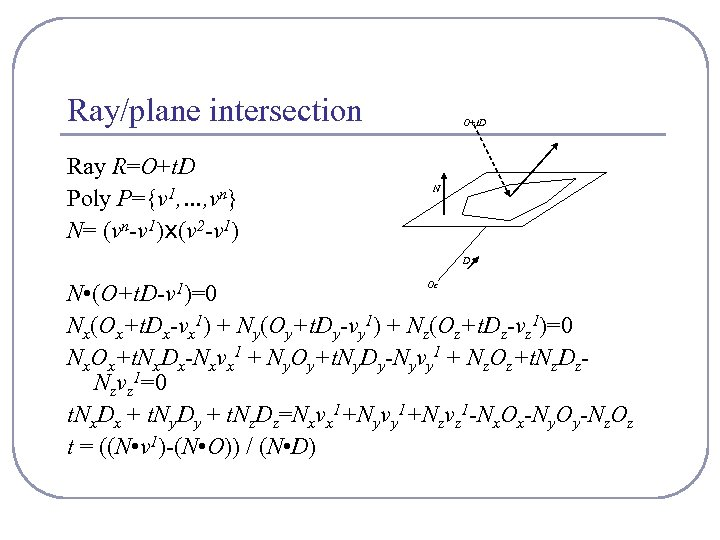Ray/plane intersection Ray R=O+t. D Poly P={v 1, …, vn} N= (vn-v 1)x(v 2 -v 1) O+t. D N D Oc N • (O+t. D-v 1)=0 Nx(Ox+t. Dx-vx 1) + Ny(Oy+t. Dy-vy 1) + Nz(Oz+t. Dz-vz 1)=0 Nx. Ox+t. Nx. Dx-Nxvx 1 + Ny. Oy+t. Ny. Dy-Nyvy 1 + Nz. Oz+t. Nz. Dz. Nzvz 1=0 t. Nx. Dx + t. Ny. Dy + t. Nz. Dz=Nxvx 1+Nyvy 1+Nzvz 1 -Nx. Ox-Ny. Oy-Nz. Oz t = ((N • v 1)-(N • O)) / (N • D)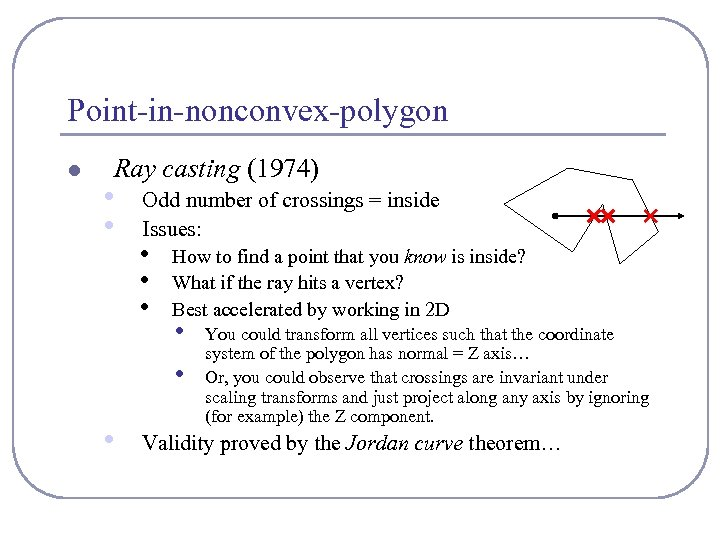Point-in-nonconvex-polygon l Ray casting (1974) • • Odd number of crossings = inside Issues: • • • How to find a point that you know is inside? What if the ray hits a vertex? Best accelerated by working in 2 D • • • You could transform all vertices such that the coordinate system of the polygon has normal = Z axis… Or, you could observe that crossings are invariant under scaling transforms and just project along any axis by ignoring (for example) the Z component. Validity proved by the Jordan curve theorem…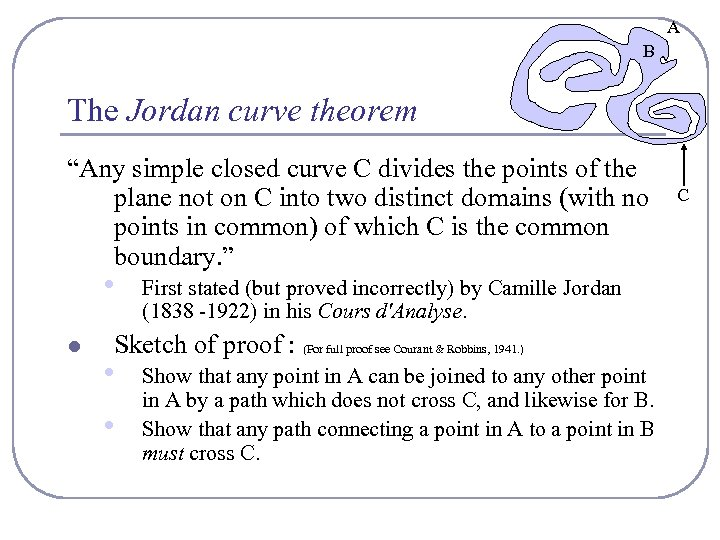A B The Jordan curve theorem “Any simple closed curve C divides the points of the plane not on C into two distinct domains (with no points in common) of which C is the common boundary. ” • l First stated (but proved incorrectly) by Camille Jordan (1838 -1922) in his Cours d'Analyse. Sketch of proof : (For full proof see Courant & Robbins, 1941. ) • • Show that any point in A can be joined to any other point in A by a path which does not cross C, and likewise for B. Show that any path connecting a point in A to a point in B must cross C. C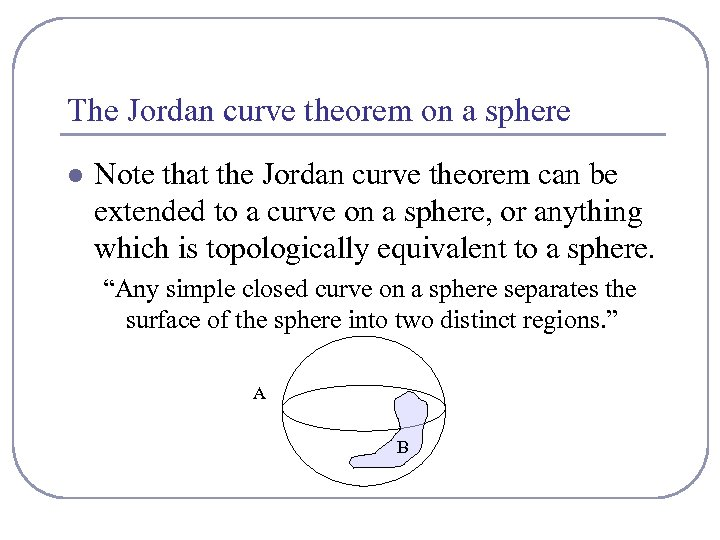The Jordan curve theorem on a sphere l Note that the Jordan curve theorem can be extended to a curve on a sphere, or anything which is topologically equivalent to a sphere. “Any simple closed curve on a sphere separates the surface of the sphere into two distinct regions. ” A B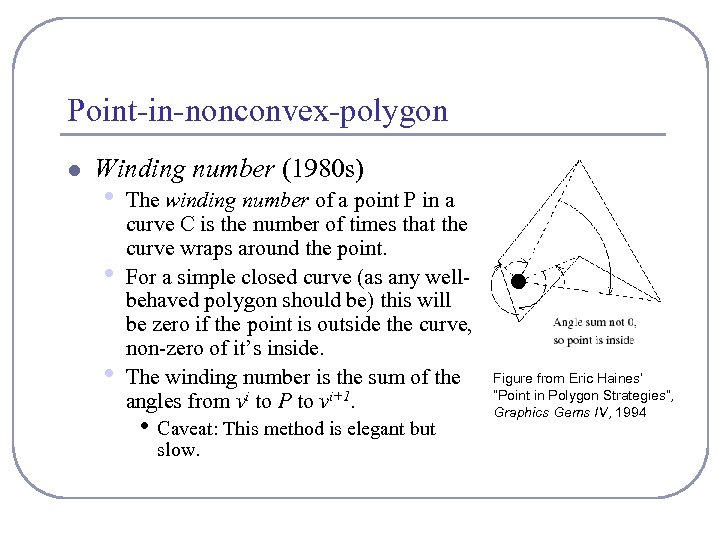Point-in-nonconvex-polygon l Winding number (1980 s) • • • The winding number of a point P in a curve C is the number of times that the curve wraps around the point. For a simple closed curve (as any wellbehaved polygon should be) this will be zero if the point is outside the curve, non-zero of it’s inside. The winding number is the sum of the angles from vi to P to vi+1. • Caveat: This method is elegant but slow. Figure from Eric Haines’ “Point in Polygon Strategies”, Graphics Gems IV, 1994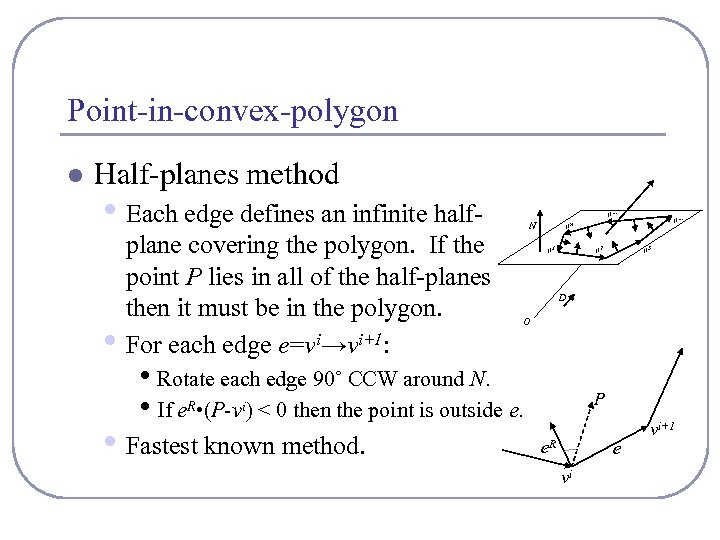Point-in-convex-polygon l Half-planes method • Each edge defines an infinite half • plane covering the polygon. If the point P lies in all of the half-planes then it must be in the polygon. For each edge e=vi→vi+1: v… N v 1 v 2 v 3 D O • Rotate each edge 90˚ CCW around N. • If e. R • (P-vi) < 0 then the point is outside e. • Fastest known method. v… vn P e. R e vi vi+1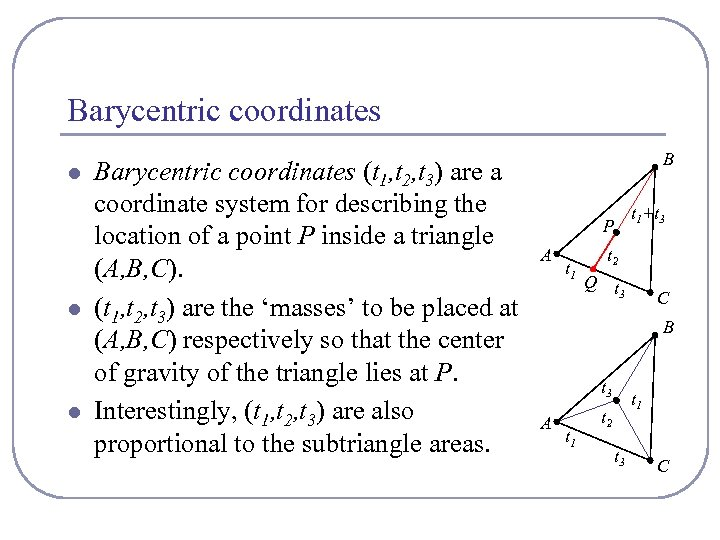Barycentric coordinates l l l Barycentric coordinates (t 1, t 2, t 3) are a coordinate system for describing the location of a point P inside a triangle (A, B, C). (t 1, t 2, t 3) are the ‘masses’ to be placed at (A, B, C) respectively so that the center of gravity of the triangle lies at P. Interestingly, (t 1, t 2, t 3) are also proportional to the subtriangle areas. B t 1+t 3 P A t 1 t 2 Q t 3 C B t 3 A t 1 t 2 t 3 C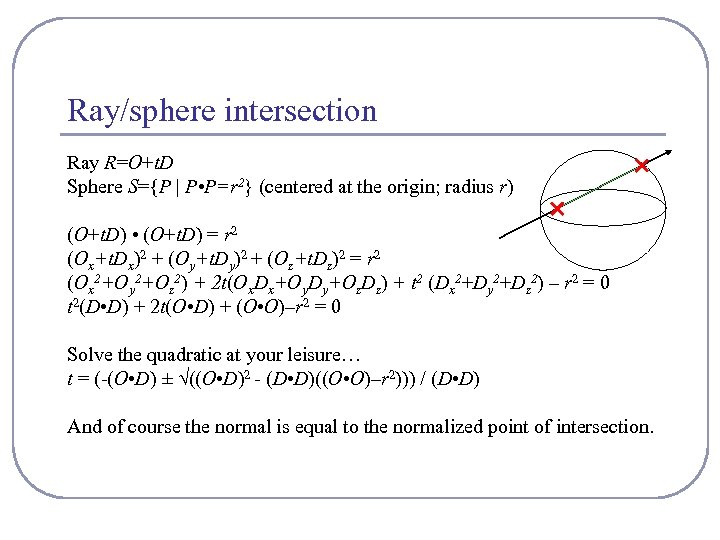Ray/sphere intersection Ray R=O+t. D Sphere S={P | P • P=r 2} (centered at the origin; radius r) (O+t. D) • (O+t. D) = r 2 (Ox+t. Dx)2 + (Oy+t. Dy)2 + (Oz+t. Dz)2 = r 2 (Ox 2+Oy 2+Oz 2) + 2 t(Ox. Dx+Oy. Dy+Oz. Dz) + t 2 (Dx 2+Dy 2+Dz 2) – r 2 = 0 t 2(D • D) + 2 t(O • D) + (O • O)–r 2 = 0 Solve the quadratic at your leisure… t = (-(O • D) ± √((O • D)2 - (D • D)((O • O)–r 2))) / (D • D) And of course the normal is equal to the normalized point of intersection.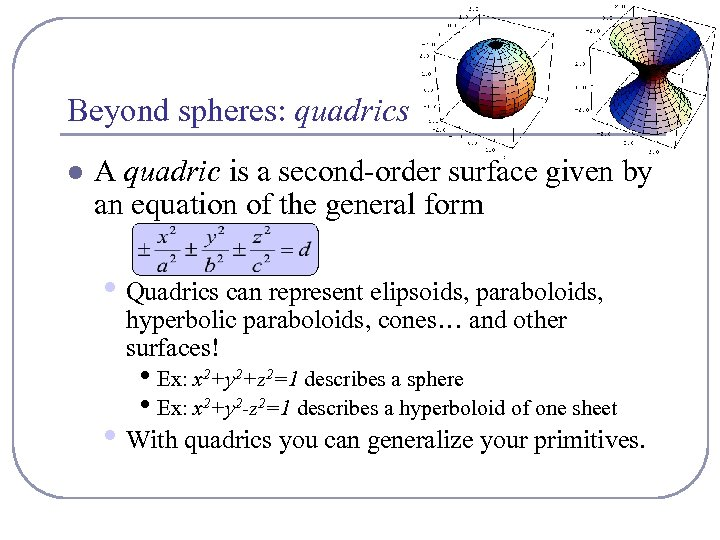Beyond spheres: quadrics l A quadric is a second-order surface given by an equation of the general form • Quadrics can represent elipsoids, paraboloids, hyperbolic paraboloids, cones… and other surfaces! • Ex: x 2+y 2+z 2=1 describes a sphere • Ex: x 2+y 2 -z 2=1 describes a hyperboloid of one sheet • With quadrics you can generalize your primitives.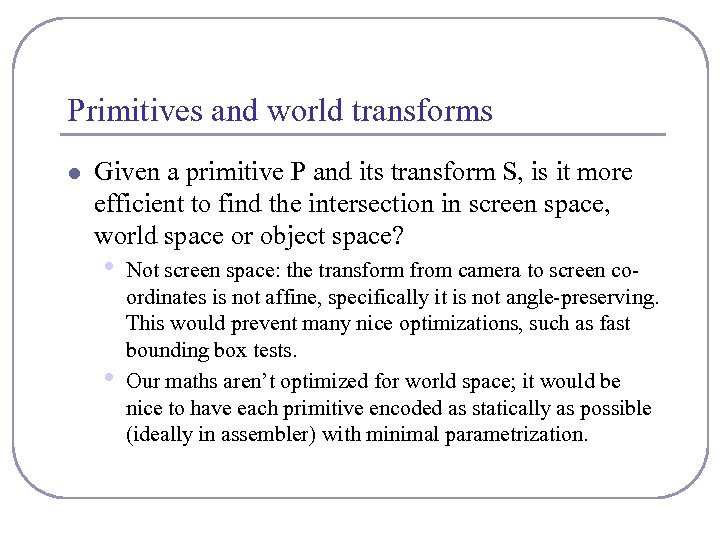Primitives and world transforms l Given a primitive P and its transform S, is it more efficient to find the intersection in screen space, world space or object space? • • Not screen space: the transform from camera to screen coordinates is not affine, specifically it is not angle-preserving. This would prevent many nice optimizations, such as fast bounding box tests. Our maths aren’t optimized for world space; it would be nice to have each primitive encoded as statically as possible (ideally in assembler) with minimal parametrization.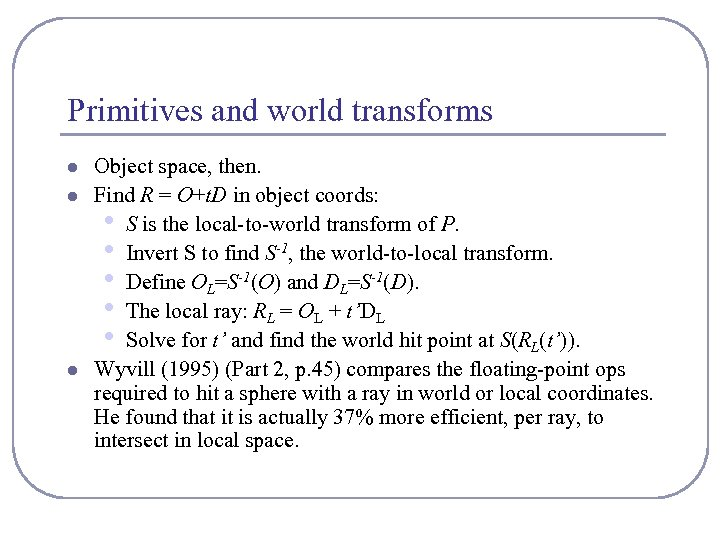Primitives and world transforms l l l Object space, then. Find R = O+t. D in object coords: • S is the local-to-world transform of P. • Invert S to find S-1, the world-to-local transform. • Define OL=S-1(O) and DL=S-1(D). • The local ray: RL = OL + t’DL • Solve for t’ and find the world hit point at S(RL(t’)). Wyvill (1995) (Part 2, p. 45) compares the floating-point ops required to hit a sphere with a ray in world or local coordinates. He found that it is actually 37% more efficient, per ray, to intersect in local space.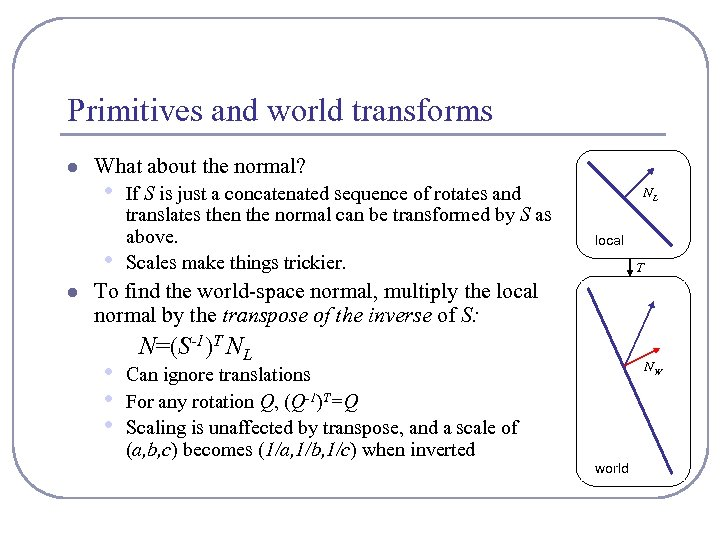Primitives and world transforms l What about the normal? • • l If S is just a concatenated sequence of rotates and translates then the normal can be transformed by S as above. Scales make things trickier. NL local T To find the world-space normal, multiply the local normal by the transpose of the inverse of S: • • • N=(S-1)T NL NW Can ignore translations For any rotation Q, (Q-1)T=Q Scaling is unaffected by transpose, and a scale of (a, b, c) becomes (1/a, 1/b, 1/c) when inverted world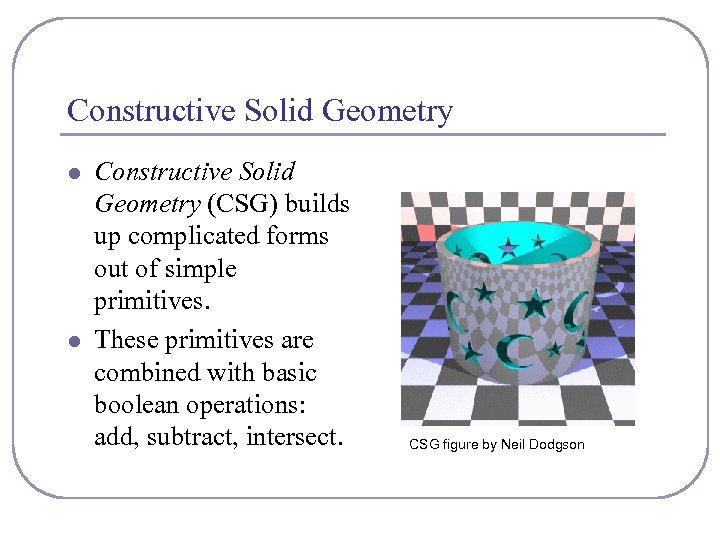Constructive Solid Geometry l l Constructive Solid Geometry (CSG) builds up complicated forms out of simple primitives. These primitives are combined with basic boolean operations: add, subtract, intersect. CSG figure by Neil Dodgson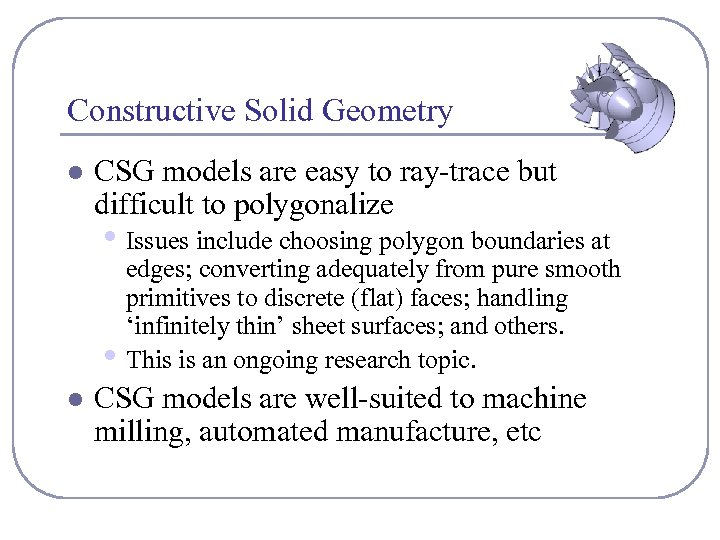Constructive Solid Geometry l CSG models are easy to ray-trace but difficult to polygonalize • Issues include choosing polygon boundaries at • l edges; converting adequately from pure smooth primitives to discrete (flat) faces; handling ‘infinitely thin’ sheet surfaces; and others. This is an ongoing research topic. CSG models are well-suited to machine milling, automated manufacture, etc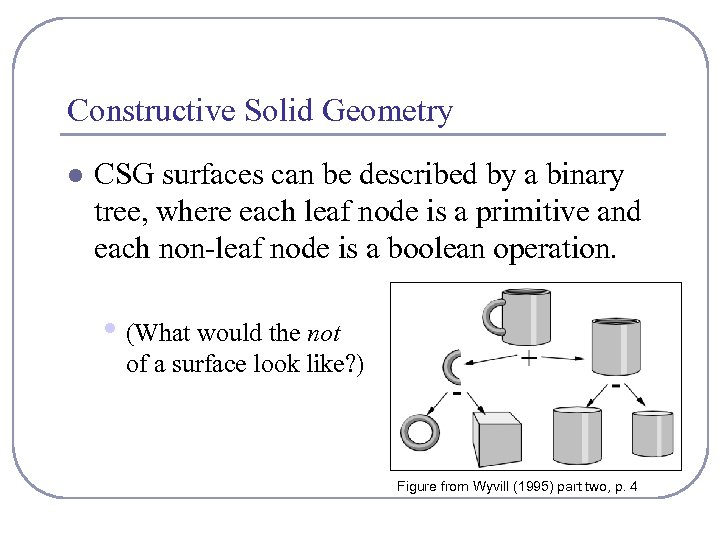Constructive Solid Geometry l CSG surfaces can be described by a binary tree, where each leaf node is a primitive and each non-leaf node is a boolean operation. • (What would the not of a surface look like? ) Figure from Wyvill (1995) part two, p. 4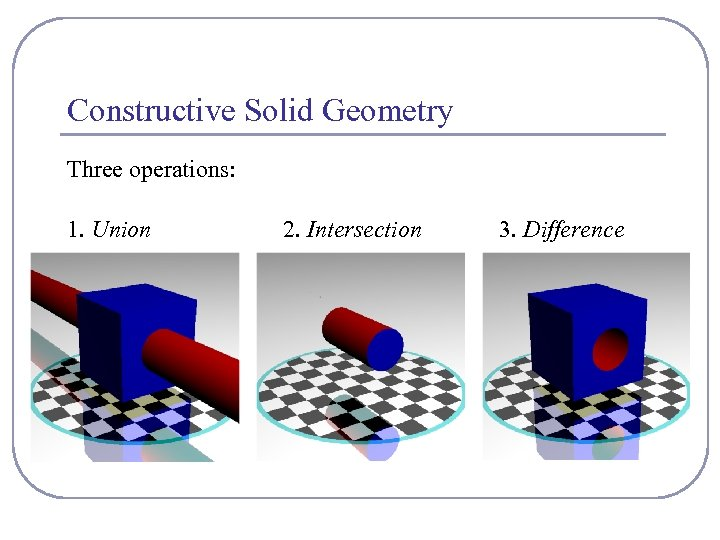Constructive Solid Geometry Three operations: 1. Union 2. Intersection 3. Difference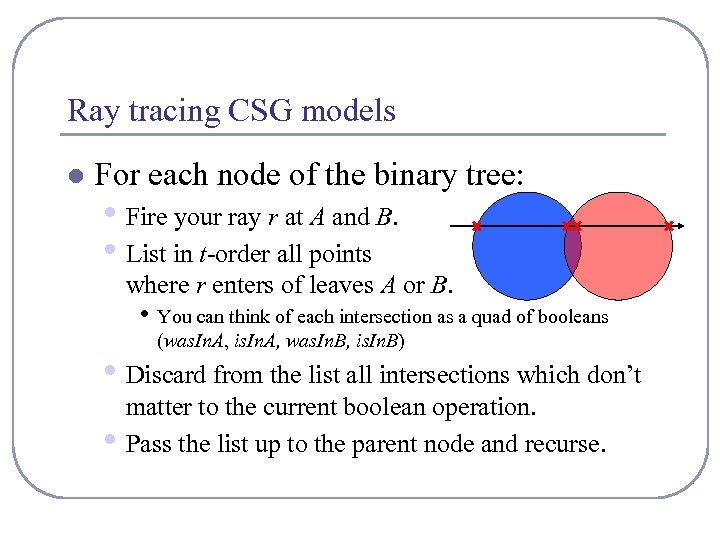Ray tracing CSG models l For each node of the binary tree: • Fire your ray r at A and B. • List in t-order all points where r enters of leaves A or B. • You can think of each intersection as a quad of booleans (was. In. A, is. In. A, was. In. B, is. In. B) • Discard from the list all intersections which don’t • matter to the current boolean operation. Pass the list up to the parent node and recurse.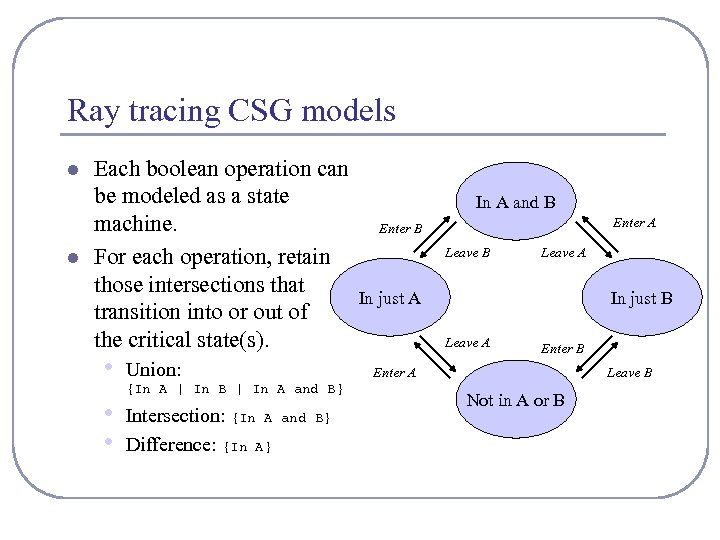Ray tracing CSG models l l Each boolean operation can be modeled as a state machine. For each operation, retain those intersections that transition into or out of the critical state(s). • • • Union: Enter A Enter B Leave A In just B Leave A Enter B Enter A {In A | In B | In A and B} Intersection: {In A Difference: {In A} In A and B} Leave B Not in A or B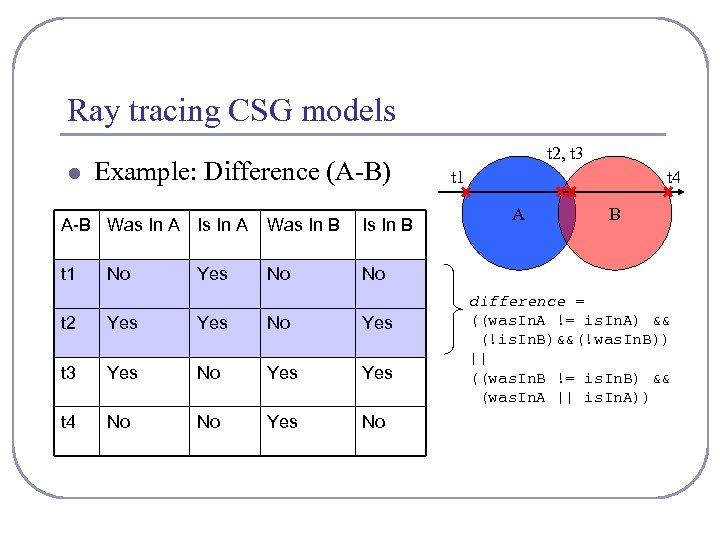Ray tracing CSG models l Example: Difference (A-B) A-B Was In A Is In A Was In B Is In B t 1 No t 2, t 3 t 1 t 4 A B No No Yes t 2 Yes No Yes t 3 Yes No Yes t 4 No No Yes No difference = ((was. In. A != is. In. A) && (!is. In. B)&&(!was. In. B)) || ((was. In. B != is. In. B) && (was. In. A || is. In. A))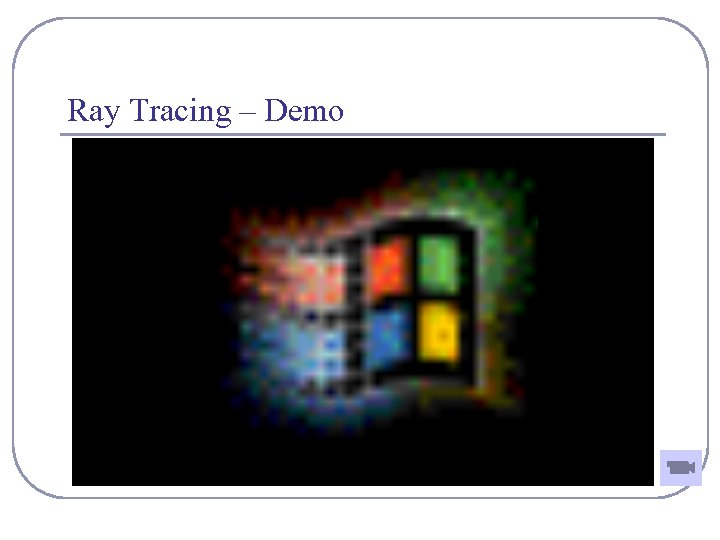Ray Tracing – Demo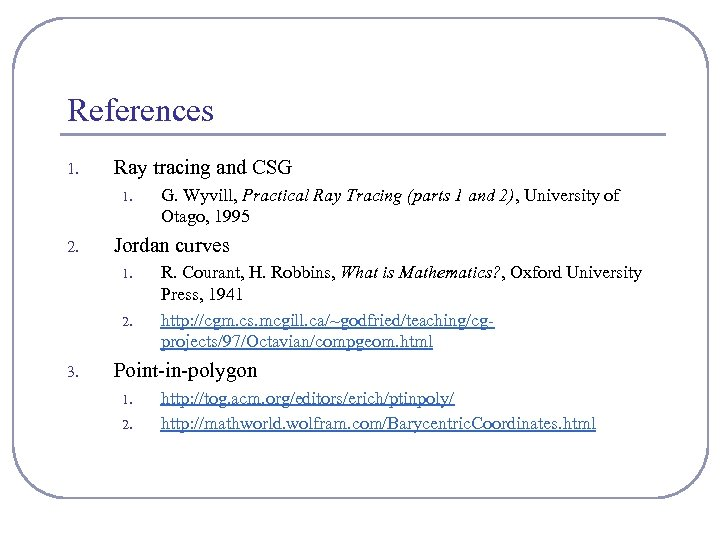References 1. Ray tracing and CSG 1. 2. Jordan curves 1. 2. 3. G. Wyvill, Practical Ray Tracing (parts 1 and 2), University of Otago, 1995 R. Courant, H. Robbins, What is Mathematics? , Oxford University Press, 1941 http: //cgm. cs. mcgill. ca/~godfried/teaching/cgprojects/97/Octavian/compgeom. html Point-in-polygon 1. 2. http: //tog. acm. org/editors/erich/ptinpoly/ http: //mathworld. wolfram. com/Barycentric. Coordinates. html